# Pair of Linear Equations in Two VariablesExercise – 3.4

1. Solve the following pair of linear equations by the elimination method and the substitution method:

(i) x + y = 5 and 2x – 3y = 4

Solution:

x + y = 5…………….(1)

2x – 3y = 4……………….(2)

In equation (1), multiplying by 2 and subtracting equation (2),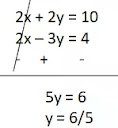Substituting the value of y in equation (1),

x + 6/5 = 5

x = 5 – (6/5)

x = 19/5

Hence, x = 19/5 and y = 6/5.

(ii) 3x + 4y = 10 and 2x – 2y = 2

Solution:

3x + 4y = 10…………….(1)

2x – 2y = 2……………….(2)

Multiplying 2 in equation (2) and adding it to equation (1),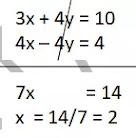Substituting the value of x in equation (2),

4 – 2y = 2

4 - 2 = 2y

y = 2/2 = 1

Hence, x = 2 and y = 1.

(iii) 3x - 5y – 4 = 0 and 9x = 2y + 7

Solution:

3x - 5y = 4…………….(1)

9x – 2y = 7……………….(2)

In equation (1), multiplying by 3 and subtracting equation (2),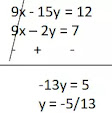Substituting the value of y in equation (1),

3x – 5×(-5/13) = 4

3x + 25/13 = 4

3x = 4 - 25/13

3x = 27/13

x = 9/13

Hence, x = 9/13 and y = –5/13.

(iv) x/2 + 2y/3 = -1 and x – y/3 = 3

Solution:

3x + 4y = -6…………….(1)

3x – y = 9……………….(2)

On subtracting equation (2) from equation (1),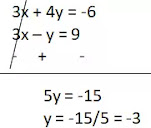Substituting the value of y in equation (2),

3x – (-3) = 9

3x = 6

x = 6/3 = 2

Hence, x = 2 and y = -3.

2. From the pair of linear equations in the following problems, and find their solutions (if they exist) by the elimination method:

(i) If we add 1 to the numerator and subtract 1 from the denominator, a fraction reduces to 1. It becomes ½ if we only add 1 to the denominator. What is the fraction?

Solution:

Let numerator = x

and denominator = y

Hence fractional number = x/y

According to the first condition,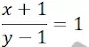x + 1 = y – 1

x – y = -2……………(1)

according to the second condition,2x = y + 1

2x – y = 1…………………(2)

On multiplying equation (1) by 2 and subtracting equation (2),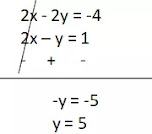Substituting the value of y in equation (2),

2x – 5 = 1

2x = 6

x = 3

Hence the fractional number = x/y = 3/5.

(ii) Five years ago, Nuri was thrice as old as Sonu. Ten years later, Nuri will be twice as old as Sonu. How old are Nuri and Sonu?

Solution:

Let Nuri's present age = x years

And present age of Sonu = y years

five years ago,

Nuri's age = x - 5 years

Sonu's age = y - 5 years

According to Question,

x – 5 = 3(y – 5)

x – 5 = 3y - 15

x – 3y = -10……………..(1)

Ten years later,

Nuri's age = x + 10 years

Sonu's age = y + 10 years

According to Question,

x + 10 = 2(y + 10)

x + 10 = 2y + 20

x – 2y = 10………………(2)

Subtracting equation (2) from equation (1),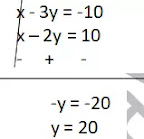Substituting the value of y in equation (2),

x – 2×20 = 10

x = 50

Hence present age of Nuri = 50 years and present age of Sonu = 20 years.

(iii) The sum of the digits of a two-digit number is 9. Also, nine times this number is twice the number obtained by reversing the order of the digits. Find the number.

Solution:

Let unit's digit = x

tens digit = y

So number = 10y + x (Multiplyed y by 10 because the number is two digit e.g.– if y = 1, x = 0 then the number 10 + 0 = 10 which is two digit)

According to the question the sum of the digits of the number is 9. so,

x + y = 9………….(1)

Number formed by reversing the digits of the number = 10x + y

According to Question,

9(number) = 2(number formed by reversing)

9(10y + x) = 2(10x + y)

90y + 9x = 20x + 2y

11x – 88y = 0

x – 8y = 0…………………(2)

Subtracting equation (2) from equation (1),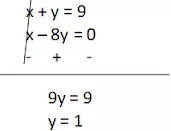Substituting the value of y in equation (1),

x + 1 = 9

x = 8

Hence the number = 10y + x = 10×1 + 8 = 18.

(iv) Meena went to a bank to withdraw ₹2000. She asked the cashier to give her ₹50 and ₹100 notes only. Meena got 25 notes in all. Find how many notes of ₹50 and ₹100 she received.

Solution:

Let the number of ₹50 notes = x

and number of ₹100 notes = y

The total number of notes is 25.

Therefore,

x + y = 25………….(1)

Total amount of ₹50 notes and ₹100 notes = ₹2000 therefore,

50x + 100y = 2000

x + 2y = 40……………..(2)

Subtracting equation (2) from equation (1),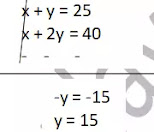Substituting the value of y in equation (1),

x + 15 = 25

x = 10

Hence, number of ₹50 notes = 10 and ₹100 notes = 15.

(v) A lending library has a fixed charge for the first three days and an additional charge for each day thereafter. Saritha paid ₹27 for a book kept for seven days, while Susy paid ₹21 for the book she kept for five days. Find the fixed charge and the charge for each extra day.

Solution:

Let the fixed charge for the first three days = x

and rent for each additional day = y

Sarita paid ₹27 to keep a book for seven days so,

x + 4y = 27……………(1)

Susy paid ₹21 to keep a book for five days so,

x + 2y = 21……………..(2)

Subtracting equation (2) from equation (1),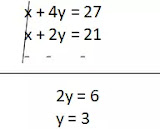Substituting the value of y in equation (1),

x + 4×3 = 27

x = 15

Hence, fixed charge for first three days = ₹ 15 and for each additional day = ₹ 3.

Download Class10 NCRT Pair of Linear Equations in Two Variables Exercise – 3.4 pdf